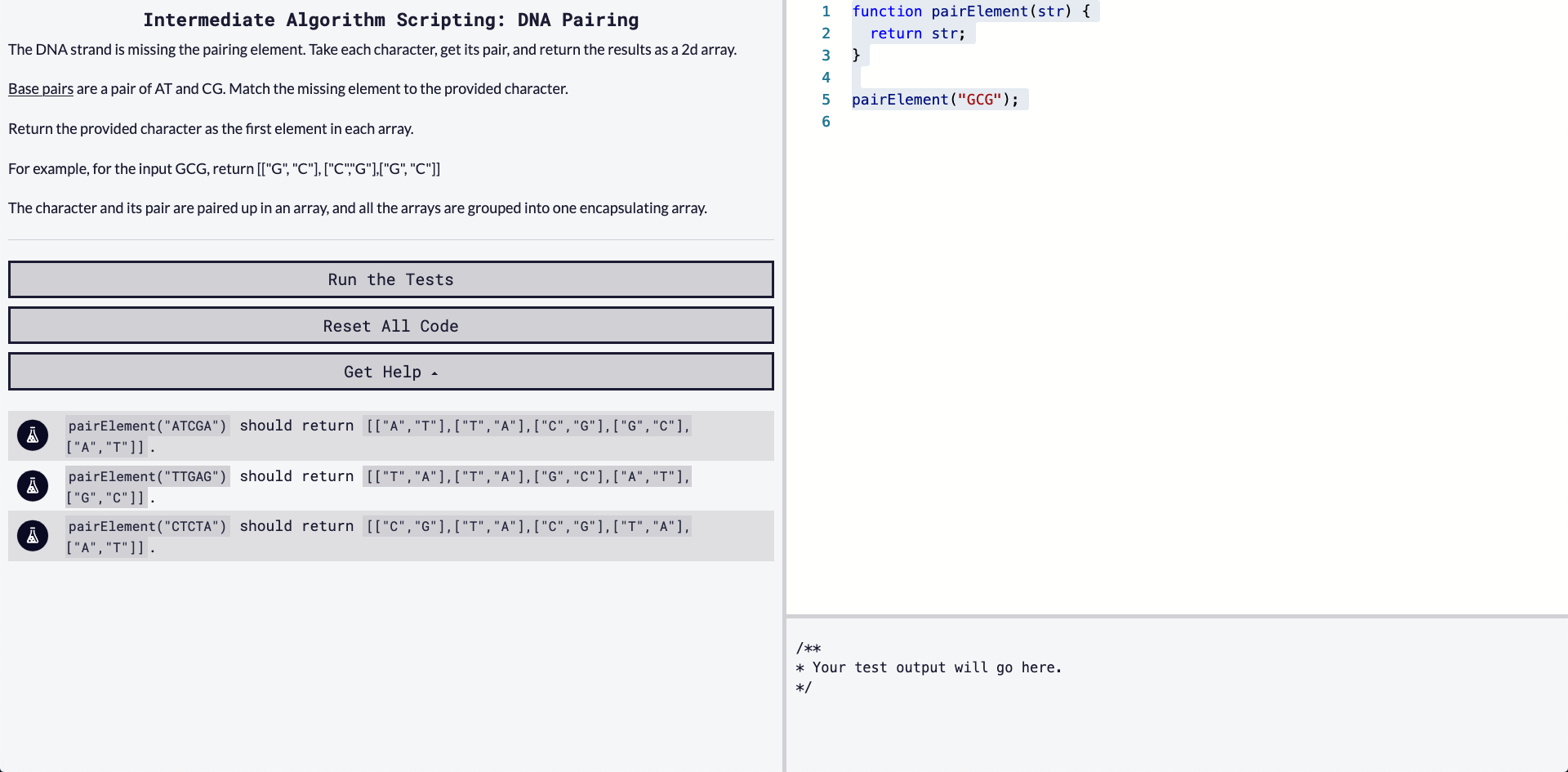## DEV Community is a community of 722,735 amazing developers

We're a place where coders share, stay up-to-date and grow their careers.

Viren B

Posted on • Originally published at virenb.cc

# Solving "DNA Pairing" / freeCodeCamp Algorithm ChallengesLet's solve freeCodeCamp's intermediate algorithm scripting challenge, 'DNA Pairing'.

### Starter Code

``````function pairElement(str) {
return str;
}

pairElement("GCG");
``````

### Instructions

The DNA strand is missing the pairing element. Take each character, get its pair, and return the results as a 2d array.

Base pairs are a pair of AT and CG. Match the missing element to the provided character.

Return the provided character as the first element in each array.

For example, for the input GCG, return [["G", "C"], ["C","G"],["G", "C"]]

The character and its pair are paired up in an array, and all the arrays are grouped into one encapsulating array.

### Test Cases

``````pairElement("ATCGA") should return [["A","T"],["T","A"],["C","G"],["G","C"],["A","T"]].
pairElement("TTGAG") should return [["T","A"],["T","A"],["G","C"],["A","T"],["G","C"]].
pairElement("CTCTA") should return [["C","G"],["T","A"],["C","G"],["T","A"],["A","T"]].
``````

# Our Approach

• We have one input, a string.
• We must return an array (a 2D array).
• We have base pairs which we must evaluate with the input string. We have to create an array and add appropriate pairs/arrays.

Hey! At least no more RegEx!

So after reading a little more about base pairs, if we come across a certain letter, we will have to find its partner, and insert that pair into our new array.

My first action is to create an empty array to house all the pairs we will have to make.

`let pairs = [];`

Before we dive into to the challenge further, let's just quickly figure how the pairing works -

• 'A' = ['A', 'T']
• 'T' = ['T', 'A']
• 'C' = ['C', 'G']
• 'G' = ['G', 'C']

So we have four cases (or for if/elses to evaluate). As the example in the instructions mentions, a test case would be

`str = 'GCG', return [["G", "C"], ["C","G"],["G", "C"]]`

I figure the best way to evaluate `str`, since its a string, is to `split('')` into an array. Then we can use a method to loop over each case. I think using `map()` is a good way to go about it.

``````str = 'GCG';
str.split('');
// Array(3) [ "G", "C", "G" ]
``````

So now, using `map()`, we can evaluate each item ('G', 'C', etc.) and see if its the correct case, we can pair it with the correct pairing in an array and push the pair into our `pairs` array (which is empty for now).

``````["G","C","G"].map(elem => {
switch (elem) {
case 'C':
pairs.push(['C', 'G'])
break;
case 'G':
pairs.push(['G', 'C'])
break;
....
}
})
``````

Another way which would work is an if/else statement.

Remember to `return pairs` (`pairs` being the new array)!

# Our Solution

``````// Two Solutions - 1) if/else, 2) switch

function pairElement(str) {
let pairs = [];
str.split('').map(elem => {
if (elem === 'G') {
pairs.push(['G', 'C'])
}
else if (elem === 'C') {
pairs.push(['C', 'G'])
}
else if (elem === 'A') {
pairs.push(['A', 'T'])
}
else if (elem === 'T') {
pairs.push(['T', 'A'])
}
})
return pairs;
}

// Second solution
function pairElement(str) {
let pairs = [];
const strArr = str.split("");
strArr.map(elem => {
switch (elem) {
case 'C':
pairs.push(['C', 'G']);
break;
case 'G':
pairs.push(['G', 'C']);
break;
case 'A':
pairs.push(['A', 'T']);
break;
case 'T':
pairs.push(['T', 'A']);
break;
}
})
return pairs;
}

pairElement("GCG");
``````